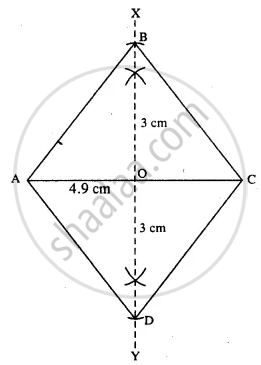# Construct a Rhombus Abcd, If ; Diagonal Ac = 4.9 Cm and Diagonal Bd = 6 Cm. - Mathematics

Sum

Construct a rhombus ABCD, if ;

diagonal AC = 4.9 cm and diagonal BD = 6 cm.

#### SolutionSteps :

1. Draw AC = 4.9 cm.
2. Draw perpendicular bisector XY of AC.
3. Cut OB = OD = 3 cm (half the diagonal 6 cm)
4. Join AB, BC, AD, and CD.
Thus ABCD is the required rhombus.
Concept: Construction of Rhombus
Is there an error in this question or solution?
Chapter 18: Constructions - Exercise 18 (D) [Page 211]

#### APPEARS IN

Selina Concise Mathematics Class 8 ICSE
Chapter 18 Constructions
Exercise 18 (D) | Q 4.6 | Page 211
Share